# (1 point) Find y as a function of t if y" – 11y' + 24y =...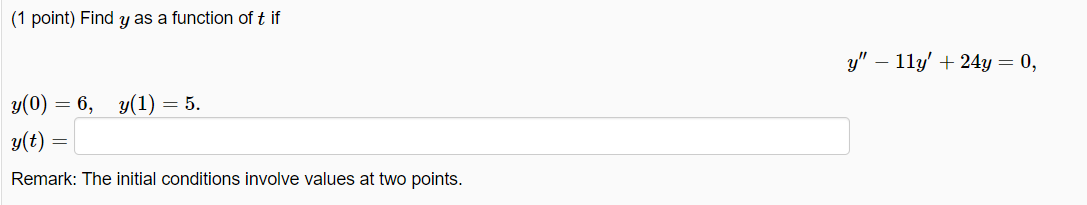(1 point) Find y as a function of t if y" – 11y' + 24y = 0, y(0) = 6, y(1) = 5. y(t) Remark: The initial conditions involve values at two points.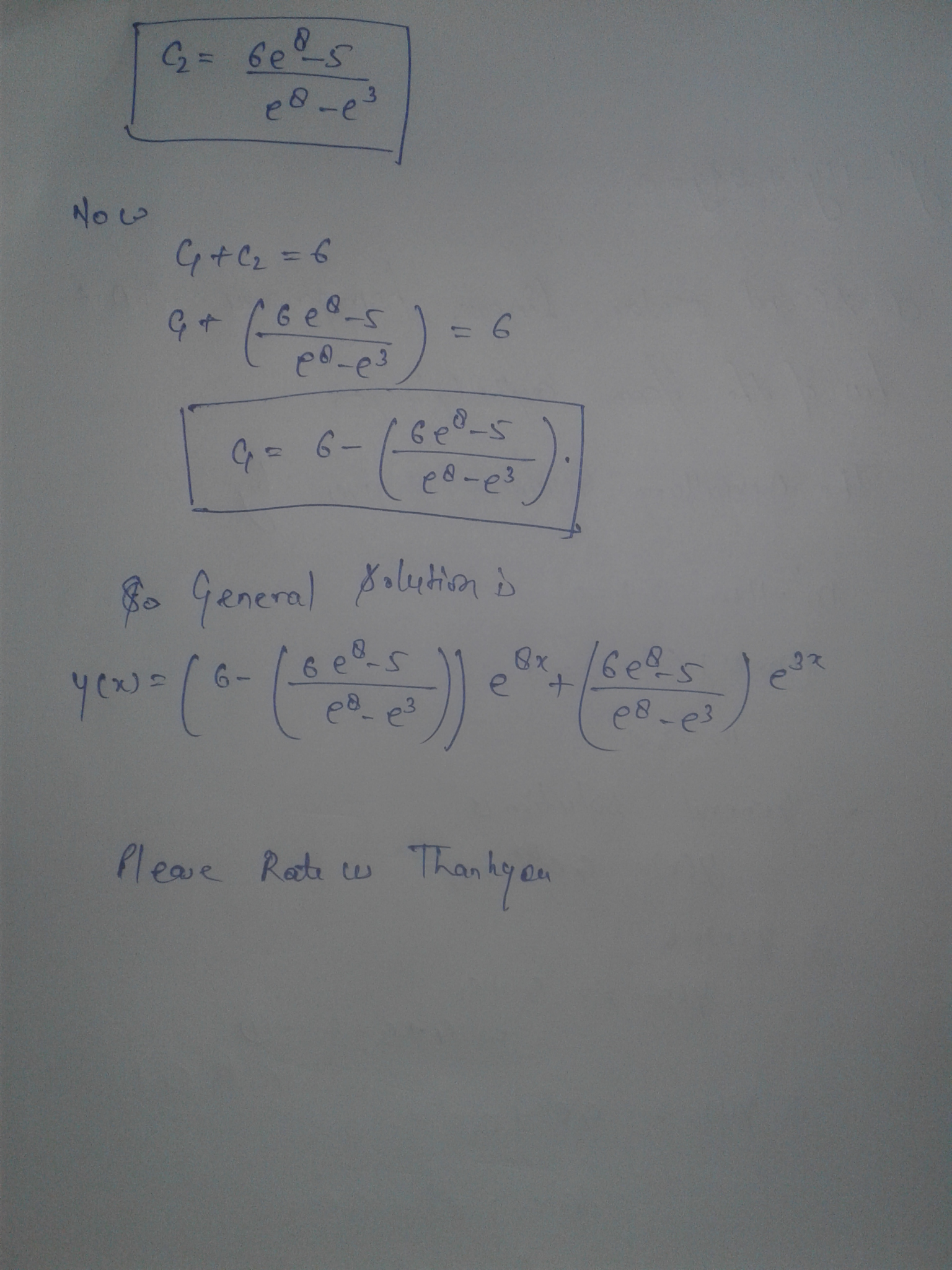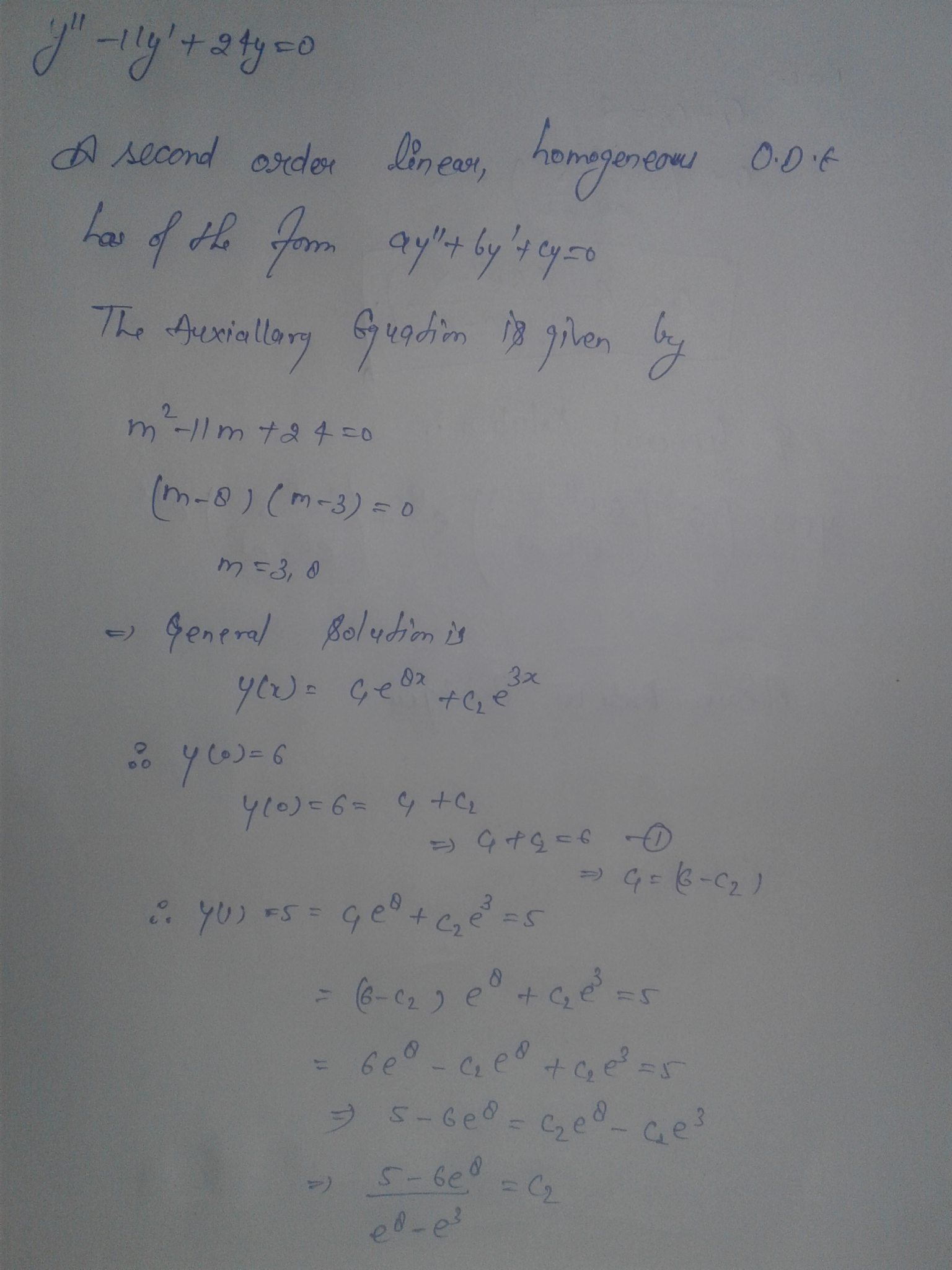##### Add Answer of: (1 point) Find y as a function of t if y" – 11y' + 24y =...
Similar Homework Help Questions
• ### (1 point) Find y as a function of lif y" - 11y +24y = 0 y(0)...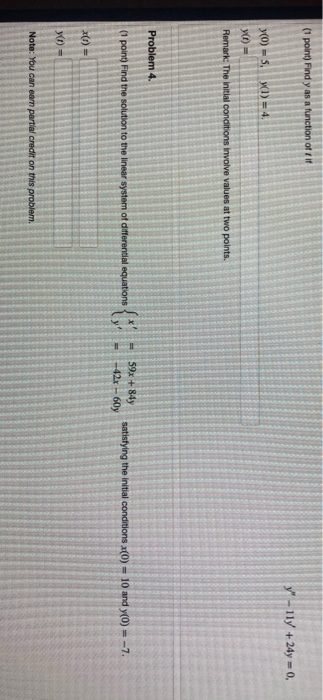(1 point) Find y as a function of lif y" - 11y +24y = 0 y(0) - S WI) = 4 W = Remark: The initial conditions involve values at two points. Problem 4. (1 point) Find the solution to the linear system of differential equations 59x +84 -42x - 607 satisfying the initial conditions (0) = 10 and y(0) -7. = X(t) = y = Note: You can earn partial credit on this problem.

• ### (1 point) Find y as a function of t if y-8y = 0, (0) - 6,...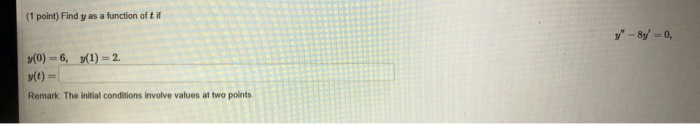(1 point) Find y as a function of t if y-8y = 0, (0) - 6, (1) = 2. y(t) Remark The initial conditions involve values at two points

• ### (1 point) Find y as a function of tif y" - 16y=0, y(0) = 5, y(1)...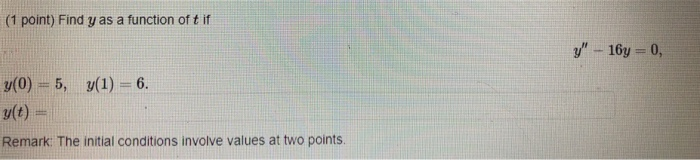(1 point) Find y as a function of tif y" - 16y=0, y(0) = 5, y(1) = 6. s(t) = Remark. The initial conditions involve values at two points

• ### Problem 3. (1 point) Find y as a function of tif y" + 5y - 14y...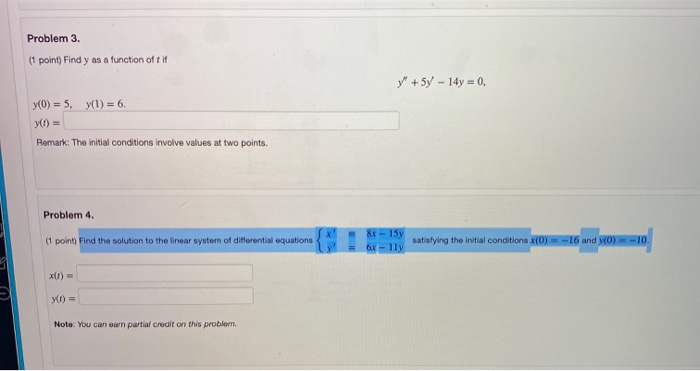Problem 3. (1 point) Find y as a function of tif y" + 5y - 14y = 0, y(0) = 5, y(1) = 6, y) = Remark: The initial conditions involve values at two points. Problem 4. (1 point) Find the solution to the linear system of differential equations 8x - 15y 6x-lly satisfying the initial conditions x(0) = -16 and yo) = -10 x(t) = Note: You can earn partial credit on this problem

• ### Problem 3. (1 point) Find y as a function of tif y" - 7 - 8y...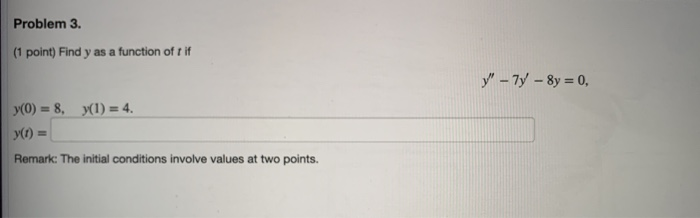Problem 3. (1 point) Find y as a function of tif y" - 7 - 8y = 0, y(O) = 8, y(1) = 4. y) = Remark: The initial conditions involve values at two points.a) Find y as a function of t ify−2y=0y(0)=7y(1)=6y(t)=Remark: The initial conditions involve values at two points.======b) Find y as a function of tif5y+29y=0y(0)=8y(0)=5y(t)=

• ### STRUGGLING WITH THESE TWO SETS OF PROBLEMS ID APPREACIATE THE CORRECT ANSWERS THANKS (1 point) If...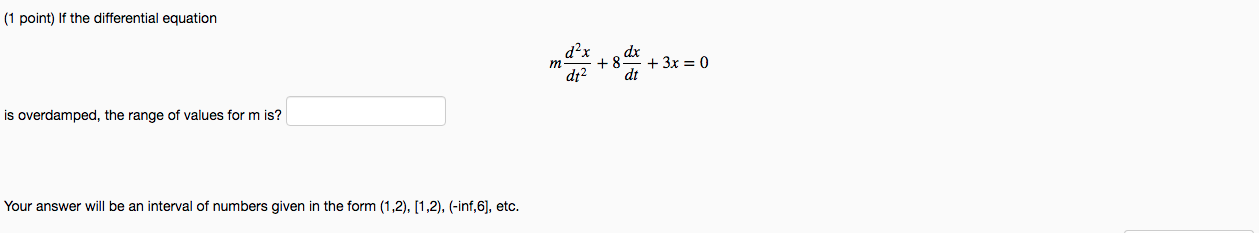STRUGGLING WITH THESE TWO SETS OF PROBLEMS ID APPREACIATE THE CORRECT ANSWERS THANKS (1 point) If the differential equation m dax + 8 dx dt2 dt + 3x = 0 is overdamped, the range of values form is? Your answer will be an interval of numbers given in the form (1,2), (1,2), (-inf,6), etc. (1 point) Find y as a function of t if y" - 6y + 8y = 0, y(O) = 5, y(1) = 4. yt) = Remark:...

• ### Rer Your answer will be an interval of numbers given in the form (1,2), (1,2), (-inf,6],...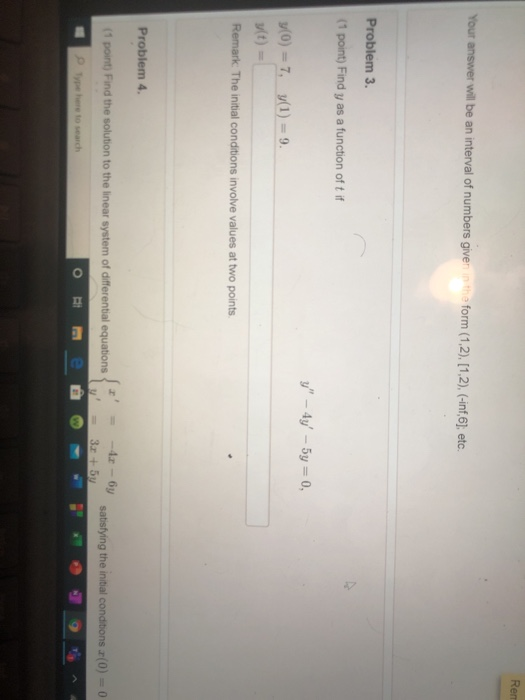Rer Your answer will be an interval of numbers given in the form (1,2), (1,2), (-inf,6], etc. Problem 3. (1 point) Find y as a function of t if y" – 4y' - 5y = 0, y(0) = 7, 3(1) = 9. u(t) Remark. The initial conditions involve values at two points. Problem 4. (1 point) Find the solution to the linear system of differential equations -42 - by 3.5 satisfying the initial conditions (0) = 0 Type here to...

• ### I AM REALLY STRUGGLING ON THIS PROBLEM PLEASE HELP ME CORRECT AND NEAT WORK IS MUCH...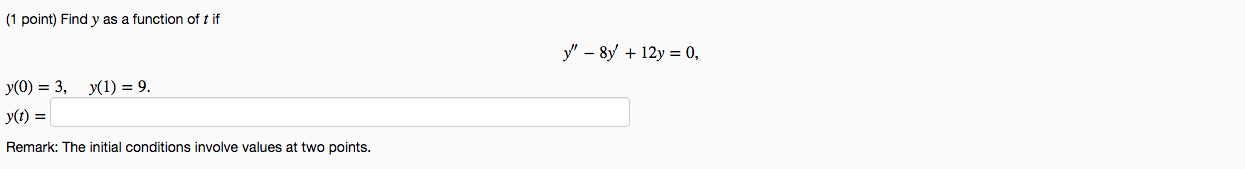I AM REALLY STRUGGLING ON THIS PROBLEM PLEASE HELP ME CORRECT AND NEAT WORK IS MUCH APPRECIATED THANKS (1 point) Find y as a function of t if y" - 8y + 12y = 0, y(0) = 3, y(1) = 9. yt) = Remark: The initial conditions involve values at two points.

• ### find Y1=, Y2=, and W(t)= (1 point) Find the function yi of t which is the...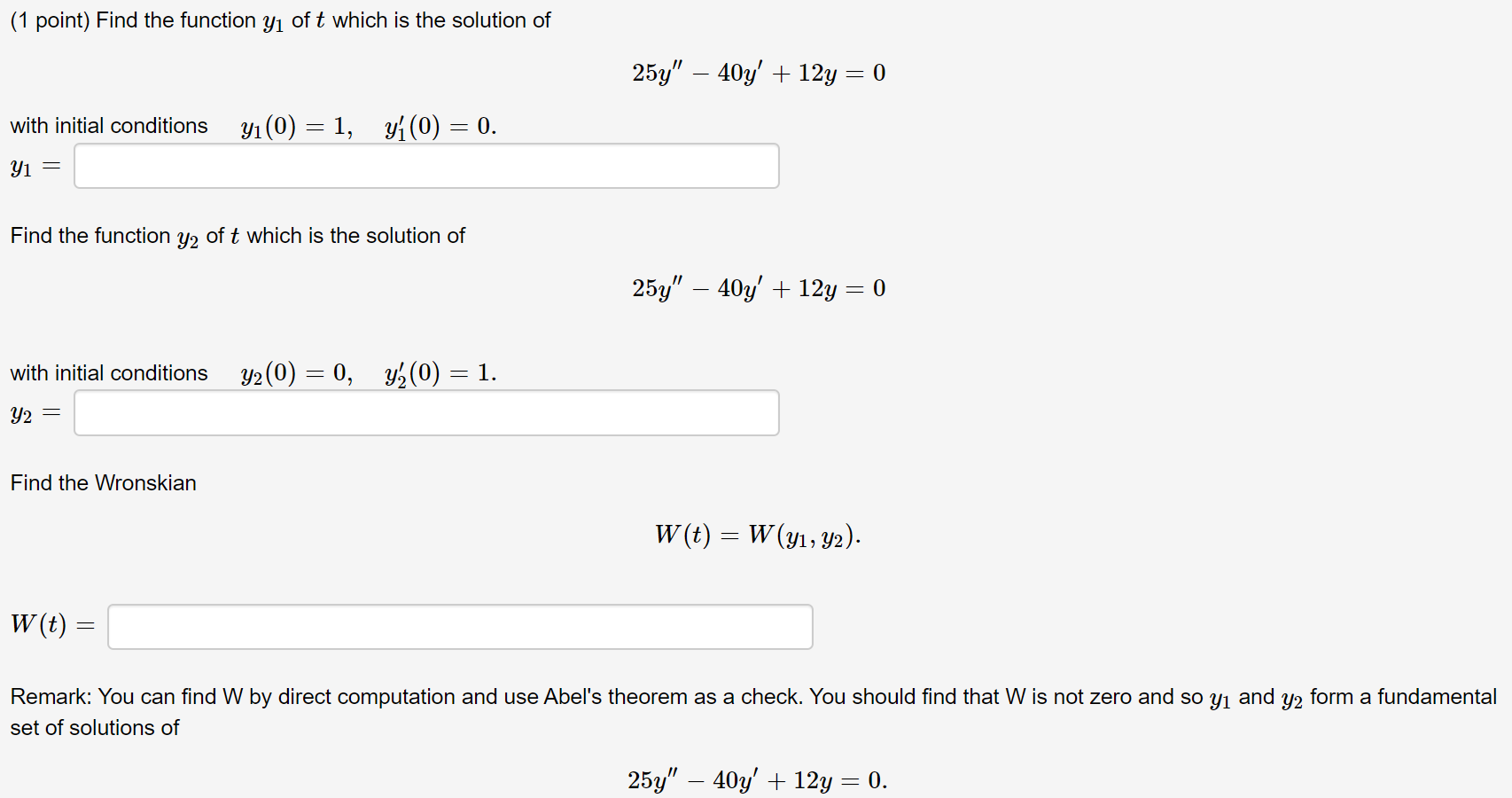find Y1=, Y2=, and W(t)= (1 point) Find the function yi of t which is the solution of 25y" – 40y' + 12y = 0 y(0) = 1, yf(0) = 0. with initial conditions Yi = Find the function y2 of t which is the solution of 25y" – 40y' + 12y = 0 with initial conditions Y2 = Find the Wronskian W(t) = W(y1, y2). W(t) = Remark: You can find W by direct computation and use Abel's theorem...

Free Homework App